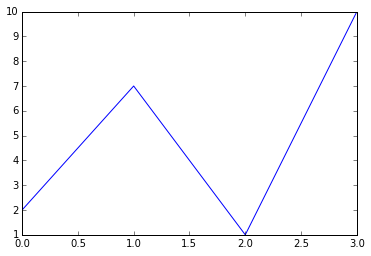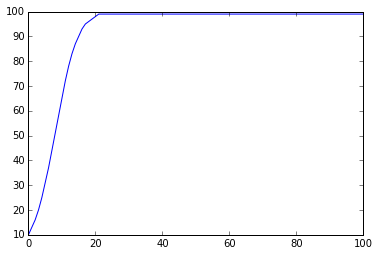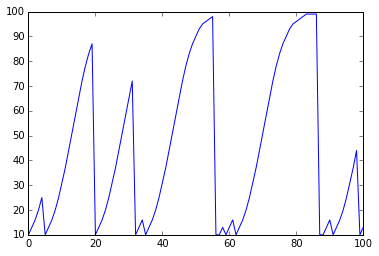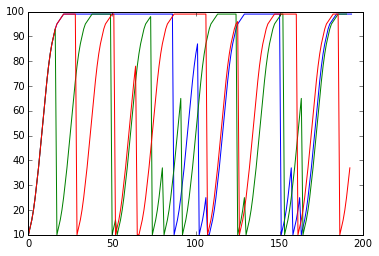# Scientific Programming I¶

### Justin Kitzes¶

Thanks to Greg Wilson and Matt Davis for ideas.

## 1. Individual things¶

The most basic component of any programming language are "things", also called variables or in some languages such as Python, objects.

The most common basic "things" in Python are integers, floats, strings, booleans, and some special objects of various types. We'll meet many of these as we go through the lesson.

TIP: To run the code in a cell quickly, press Ctrl+Enter. To run the code in a cell and then proceed to the next cell, press Ctrl+Shift. The Help menu above lists more shortcuts under the Keyboard Shortcuts menu item. You can also use the buttons under the menu to delete cells (use the Cut button), create new ones, move cells, etc.

In :
# A thing
2

Out:
2
In :
# Use print to show multiple things in the same cell
print(2)
print('hello')  # Both single and double quotes are fine

2
hello

In :
# Things can be stored as variables
a = 2
b = 'hello'
c = True  # This is case sensitive
print(a, b, c)

2 hello True

In :
# We can use type to determine what kind of thing we have
print(type(a))
print(type(b))
print(type(b))

<class 'int'>
<class 'str'>
<class 'str'>


## 2. Commands that operate on things¶

While things are great, things by themselves aren't that much use to us. Right away, we'd like to start doing stuff, that is peforming operations, with various things. There are three common means of performing an operation on a thing.

### 2.1 Use an operator¶

All of the basic math operators work like you think they should for numbers. They can also do some useful operations on other things, like strings. There are also boolean operators that compare quantities and give back a bool variable as a result.

In :
# Standard math operators work as expected on numbers
a = 2
b = 3
print(a + b)
print(a * b)
print(a ** b)  # You don't want ^

5
6
8

In :
# There are also operators for strings
print('hello' + 'world')
print('hello' * 3)
#print('hello' / 3)

helloworld
hellohellohello

In :
# Boolean operators compare two things
a = (1 > 3)
b = (3 == 3)
print(a)
print(b)
print(a or b)
print(a and b)

False
True
True
False


### 2.2 Use a function¶

These will be very familiar to anyone who has programmed in any language, and work like you would expect.

In :
# There are thousands of functions that operate on things
print(type(3))  # We've already met print and type
print(type('hello'))
print(len('hello'))
print(round(3.3))

<class 'int'>
<class 'str'>
5
3


TIP: To find out what a function does, you can type it's name and then a question mark to get a pop up help window. The window will describe the function and also tell you what input parameters it takes. Parameters in square brackets are optional.

In :
#round?
round(3.14159, 2)

Out:
3.14

TIP: Many useful functions are not built in to core Python, but are found in external scientific packages. These need to be imported into your Python notebook (or program) before they can be used. In addition to functions, these external packages also often include new types of things, such as numeric arrays and data tables. We'll meet several of the most important scientific packages in the next lesson. When these packages are imported into Python, we usually refer to them as modules.

In :
# Import the random module and use a function from it
# After importing a module, use a '.' to look inside of it
import random as rnd
rnd.random()

Out:
0.5424361633616448

### 2.3 Use a method¶

Before we get any farther into the Python language, we have to say a word about "objects". We will not be teaching object oriented programming in this workshop, but you will encounter objects throughout Python (in fact, even seemingly simple things like ints and strings are actually objects in Python).

In the simplest terms, you can think of an object as a small bundled "thing" that contains within itself both data and functions that operate on that data. For example, strings in Python are objects that contain a set of characters and also various functions that operate on the set of characters. When bundled in an object, these functions are called "methods". The reason to bundle a function up as a method, instead of making it free floating, is that it makes it clear what kind of variable the function can operate on.

Instead of the "normal" function(arguments) syntax, methods are called using the syntax variable.method(arguments). Think of the '.' as short for "look inside".

In :
# A string is an object
a = 'hello, world'

In :
# Strings have many methods
print(a.capitalize())
print(a.replace('l', 'X'))

Hello, world
heXXo, worXd


### EXERCISE 1 - Introducing logistic growth¶

Throughout this lesson, we will successively build towards a program that will calculate the logistic growth of a population of bacteria in a petri dish (or bears in the woods, if you prefer). Logistic growth produces a classic S shaped curve in which a population initially grows very fast, then slows down over time until it reaches a steady state value known as the carrying capacity.

For example, when there are only few bears in the woods and lost of food, they reproduce very fast. As the woods get full of bears and food gets harder to find, growth slows until the number of bears just balances with the amount of food (and hunters) in the woods, at which point the population stops growing.

A commonly used discrete time equation for logistic population growth is

$$n_{t+1} = n_{t} + r n_{t} (1 - n_{t} / K)$$

where $n_t$ is the population size at time $t$, $r$ is the maximum net per capita growth rate, and $K$ is the carrying capacity of the dish/woods.

To get started, write Python expressions that do the following:

1. Create variables for r, K, and n0 (the population at time $t = 0$), setting these equal to 0.3, 100, and 10, respectively.
2. Create the variable n1 and calculate it's value. Do the same for n2.
3. Check the type of n2 - what is it?

Bonus

1. Figure out how to test whether n2 is an integer (a mathematical integer, not necessarily whether it is an integer type) (HINT: look at the methods of n2 by typing n2. and pressing tab.)
2. Modify your calculations for n1 and n2 so that these values are rounded to the nearest integer. When you do so, you might notice that your answer to Bonus #1 above stops working --- why?
In :
r = 0.3
K = 100
n0 = 10

n1 = n0 + r*n0*(1 - n0/K) # round()
n2 = n1 + r*n1*(1 - n1/K) # round()

print(n0, n1, n2)
print(type(n2))

print('n2 is an integer: ', n2.is_integer())

10 12.7 16.02613
<class 'float'>
n2 is an integer:  False


## 3. Collections of things¶

Once the number of variables that you are interested in starts getting large, working with them all individually starts to get unwieldy. To help stay organized, we can use collections of things.

There are many types of collections in Python, including lists, tuples, dictionaries, and numpy arrays. Here we'll look just at the most flexible and simplest container, the list. Lists are declared using square brackets []. You can get individual elements out of a list using the syntax a[idx].

In :
# Lists are created with square bracket syntax
a = ['hi', 'hello', 'yo']
print(a)
print(type(a))

['hi', 'hello', 'yo']
<class 'list'>

In :
# Lists (and all collections) are also indexed with square brackets
# NOTE: The first index is zero, not one
print(a)
print(a)

hi
hello

In :
# Lists can be sliced by putting a colon between indexes
# NOTE: The end value is not inclusive
print(a[0:2])

# Think of this behavior as counting the "walls" between items

['hi', 'hello']

In :
# You can leave off the start or end if desired
print(a[:2])
print(a[2:])
print(a[:])
print(a[:-1])

['hi', 'hello']
['yo']
['hi', 'hello', 'yo']
['hi', 'hello']

In :
# Lists are objects, like everything else, and have methods such as append
a.append('hiya')
print(a)

a.append([1,2])
print(a)

a.pop()
print(a)

['hi', 'hello', 'yo', 'hiya']
['hi', 'hello', 'yo', 'hiya', [1, 2]]
['hi', 'hello', 'yo', 'hiya']


### EXERCISE 2 - Storing population size in a list¶

Copy your code from Exercise 1 into the box below, and do the following:

1. Modify your code so that the values of n0, n1, and n2 are stored in a list and not as separate individual variables. HINT: You can start off by declaring an empty list using the syntax n = [], and then append each new calculated value of nt to the list.
2. Get the first and last values in the list, calculate their ratio, and print out "Grew by a factor of " followed by the result.

Bonus

1. Extract the last value in two different ways: first, by using the index for the last item in the list, and second, presuming that you do not know how long the list is.
In :
######################################
# This code deletes our old variables
try: del n0, n1, n2, r, K
except: pass
######################################

r = 0.3
K = 100
n = []
n.append(10)  # Append n0 in the first location

n.append(round(n + r*n*(1 - n/K)))  # Append n1
n.append(round(n + r*n*(1 - n/K)))  # Append n2

print(n)

print("Grew by a factor of ", n/n)  # or n[-1]/n

[10, 13, 16]
Grew by a factor of  1.6


## 4. Repeating yourself¶

So far, everything that we've done could, in principle, be done by hand calculation. In this section and the next, we really start to take advantage of the power of programming languages to do things for us automatically.

We start here with ways to repeat yourself. The two most common ways of doing this are known as for loops and while loops. For loops in Python are useful when you want to cycle over all of the items in a collection, such as a list, and while loops are useful when you want to cycle for an indefinite amount of time until some condition is met.

In :
# A basic for loop - pay attention to the white space!
wordlist = ['hi', 'hello', 'bye']
for word in wordlist:
print(word + '!')

hi!
hello!
bye!

In :
# Sum all of the values in a collection using a for loop
numlist = [1, 4, 77, 3]
total = 0

for num in numlist:
total = total + num

print("Sum is", total)

Sum is 85

In :
# Sometimes we want to loop over the indexes of a collection, not just the items
print(wordlist)

length = len(wordlist)
print(length)

for i in range(length):
print(i, wordlist[i])

['hi', 'hello', 'bye']
3
0 hi
1 hello
2 bye

In :
# While loops are useful when you don't know how many steps you will need,
# and want to stop once a certain condition is met.
stp = 0
prd = 1
while prd < 100:
stp = stp + 1
prd = prd * 2
print(stp, prd)

print('Reached a product of', prd, 'at step number', stp)

1 2
2 4
3 8
4 16
5 32
6 64
7 128
Reached a product of 128 at step number 7


TIP: Once we start really generating useful and large collections of data, it becomes unwieldy to inspect our results manually. The code below shows how to make a very simple plot. We'll do more plotting later.

In :
# Jupyter notebook command to load plotting functions
%pylab inline

# Make some data and plot it
y = [2,7,1,10]
plot(y)

Populating the interactive namespace from numpy and matplotlib

Out:
[<matplotlib.lines.Line2D at 0x106a23358>]### EXERCISE 3 - Using loops to repeat calculations¶

Let's get smart about calculating our population size. Copy your code from Exercise 2 into the box below, and do the following:

1. Write a for loop to calculate and store the values of nt for 100 time steps. HINT: Use the range function to pick the number of time steps. For each step, append the new population value to a list called n. What indexing strategy can you use to get the last value of the list each time around the for loop?
2. Plot the population sizes in the list n.
3. Play around with the values of r and K and see how it changes the plot. What happens if you set r to 1.9 or 3, or values in between?

Bonus

1. Modify your code to use a while loop that will stop your calculation after the population size exceeds 80. HINT: Start a step counter i at 1, and check that the population size is less than 80 each time around the loop. Increment the step counter within the loop so that you have a record of what step the calculation stopped at.
In :
r = 0.3
K = 100
n0 = 10

n = []
n.append(n0)

for i in range(100):
n.append(round(n[-1] + r*n[-1]*(1 - n[-1]/K)))

plot(n)

Out:
[<matplotlib.lines.Line2D at 0x106acbd68>]In :
r = 0.3
K = 100
n = []
n.append(10)

i = 1
while n[-1] < 80:
n.append(round(n[i-1] + r*n[i-1]*(1 - n[i-1]/K)))
print(i, n[i])
i = i + 1

print('Ended with a population size of', n[i-1], 'at step', i-1)
print('Population sizes to this step were', n[0:i])

1 13
2 16
3 20
4 25
5 31
6 37
7 44
8 51
9 58
10 65
11 72
12 78
13 83
Ended with a population size of 83 at step 13
Population sizes to this step were [10, 13, 16, 20, 25, 31, 37, 44, 51, 58, 65, 72, 78, 83]


## 5. Making choices¶

Often we want to check if a condition is True and take one action if it is, and another action if the condition is False. We can achieve this in Python with an if statement.

TIP: You can use any expression that returns a boolean value (True or False) in an if statement. Common boolean operators are ==, !=, <, <=, >, >=.

In :
# A simple if statement
x = 3
if x > 0:
print('x is positive')
elif x < 0:
print('x is negative')
else:
print('x is zero')

x is positive

In :
# If statements can also use boolean variables
x = -1
test = (x > 0)
if test:
print('Test was true')


### EXERCISE 4 - Adding some randomness to the model¶

Let's introduce some element of randomness into our population growth model. We'll model a simple "catastrophe" process, in which a catastrophe happens in 10% of the time steps that reduces the population back down to the initial size. Copy your code from Exercise 4 into the box below, and do the following:

1. Inside your for loop, add a variable called cata, for catastrophe, that will be True if a catastrophe has occurred, and False if it hasn't. A simple way to do this is to generate a pseudorandom number between 0 and 1 using rnd.random(). Check whether this number is less than 0.1 - this check will be True 10% of the time.
2. Using your boolean variable cata, add an if statement to your for loop that checks whether cata is true in each time step. If it is true, set the population back to the initial size. Otherwise, perform the usual logistic growth calculation.
3. Plot your results. Run the cell again to see a different random population growth path.

Bonus

1. Count the number of time steps in your list n in which the population was above 50.
In :
r = 0.3
K = 100
n0 = 10

n = []
n.append(n0)

for i in range(100):
cata = (rnd.random() < 0.1)  # Random catastrophe 10% of time
if cata:
n.append(n0)
else:
n.append(round(n[-1] + r*n[-1]*(1 - n[-1]/K)))

plot(n)

Out:
[<matplotlib.lines.Line2D at 0x106d08828>]In :
above = 0
for pop in n:
if pop > 50:
above += 1

print('Population was above 50 in', above, 'out of 100 steps')

Population was above 50 in 41 out of 100 steps


## 6. Creating chunks with functions and modules¶

One way to write a program is to simply string together commands, like the ones above, in one long file, and then to run that file to generate your results. This may work, but it can be cognitively difficult to follow the logic of programs written in this style. Also, it does not allow you to reuse your code easily - for example, what if we wanted to run our logistic growth model for several different choices of initial parameters?

The most important ways to "chunk" code into more manageable pieces is to create functions and then to gather these functions into your own modules, and eventually packages. Below we will discuss how to create functions and modules. A third common type of "chunk" in Python is classes, but we will not be covering object-oriented programming in this workshop.

In :
# It's very easy to write your own functions
def multiply(x, y):
return x*y

In :
# Once a function is "run" and saved in memory, it's available just like any other function
print(type(multiply))
print(multiply(4, 3))

<class 'function'>
12

In :
# It's useful to include docstrings to describe what your function does
def say_hello(time, people):
return 'Good ' + time + ', ' + people

In :
say_hello('afternoon', 'friends')

Out:
'Good afternoon, friends'
In :
# All arguments must be present, or the function will return an error
#say_hello('afternoon')

In :
# Keyword arguments can be used to make some arguments optional by giving them a default value
# All mandatory arguments must come first, in order
def say_hello(time, people='friends'):
return 'Good ' + time + ', ' + people

In :
say_hello('afternoon')

Out:
'Good afternoon, friends'
In :
say_hello('afternoon', 'students')

Out:
'Good afternoon, students'

### EXERCISE 6 - Creating a logistic growth function¶

Finally, let's turn our logistic growth model into a function that we can use over and over again. Copy your code from Exercise 5 into the box below, and do the following:

1. Turn your code into a function called logistic_growth that takes four arguments: r, K, n0, and p (the probability of a catastrophe). Make p a keyword argument with a default value of 0.1. Have your function return the n list of population sizes.
2. In a subsequent cell, call your function with different values of the parameters to make sure it works. Store the returned value of n and make a plot from it.

Bonus

1. Refactor your function by pulling out the line that actually performs the calculation of the new population given the old population. Make this line another function called grow_one_step that takes in the old population, r, and K, and returns the new population. Have your logistic_growth function use the grow_one_step function to calculate the new population in each time step.
In :
def logistic_growth(r, K, n0, p=0.1):

n = []
n.append(n0)

for i in range(100):
cata = (rnd.random() < p)  # Random catastrophe 10% of time
if cata:
n.append(n0)
else:
n.append(round(n[-1] + r*n[-1]*(1 - n[-1]/K)))
#n.append(grow_one_step(n[-1], r, K))

return n

def grow_one_step(nold, r, K):
return round(nold + r*nold*(1 - nold/K))

In :
a1 = logistic_growth(0.3, 100, 10)
a2 = logistic_growth(0.3, 100, 10)
a3 = logistic_growth(0.3, 100, 10)

plot(a1)
plot(a2)
plot(a3)

Out:
[<matplotlib.lines.Line2D at 0x106dde160>]